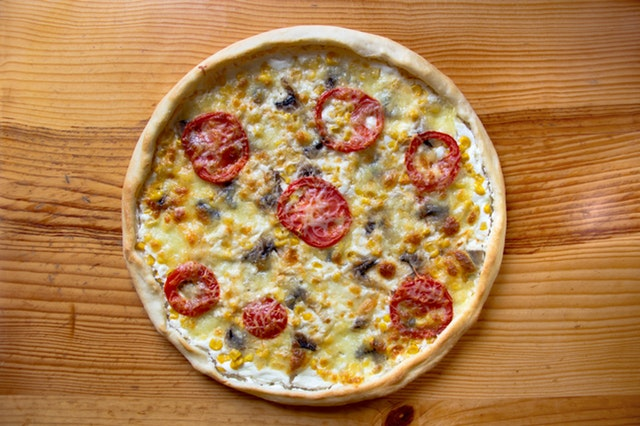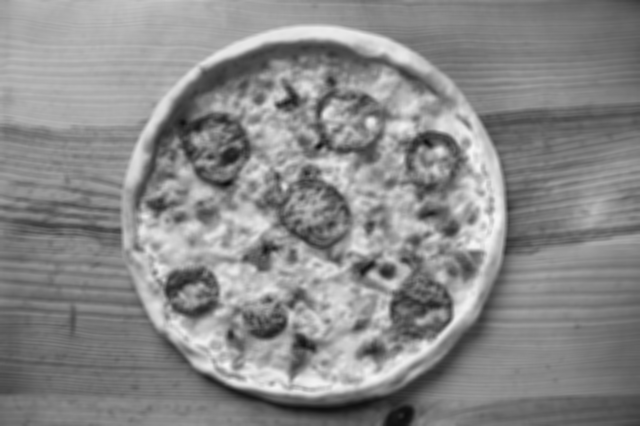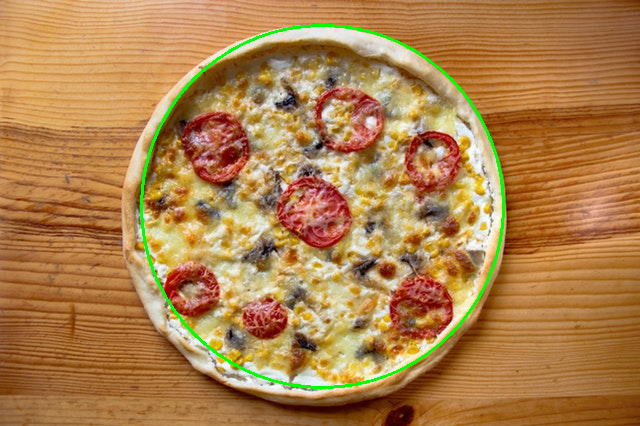# Pythonic intro to OpenCV.

Posted on pon 22 lipca 2019 in Data Science, Computer Vision

## What is OpenCV and what can you use it for?

Tons of stuff is written on OpenCV. However, it originates from C++ and with the rise of Python, its' API was developed as well. That's why when you struggle with some problem, majority of OpenCV examples will be written in C++, and it's the reason why today I am going to show you a few pythonic snippets describing:

• how can you open and get basic image information
• how can detect object on image
• how can you cut a part of image that is important to you
• how can you save your results

Today's post is a first part of a mini-project, where we explore unsupervised machine learning methods and build a classifier that checks if pizza appears on a given image.

## Pizza project description

We all love pizza, don't we? As we also love machine learning, we would like to build a classifier that checks if a given photography shows pizza on it.

How would we approach it?

1. We need to collect data
2. We need to build our classifier
3. We need to evaluate our model

Today, we are going to take care of the first point, but in coming next weeks, I will show you how can you do the remaining two. Want to stay updated?

## How can you open and get basic image info using OpenCV

Reading image in OpenCV can be done with the following lines:

``````import cv2
import numpy as np
print(img_colored.shape)
print(type(img_colored))
print(np.max(img_colored))
``````

Output:

``````(426, 640, 3) # Height, Width, Number of channels
<class 'numpy.ndarray'>
``````As you can see, every image after being read, is a numpy array. Moreover, every image is represented in BGR color space, which means that there are 426x640 of color components (blue, green and red). These components can take value from 0 to 255. So to explain it in plain words, for computer, our picture looks like this:

``````<------------ 640 columns ---------------->

+-----------+-----+-----------+-----------+
| [0,2,189] | ... | [0,2,189] | [0,2,189] |   |
+-----------+-----+-----------+-----------+   |
| [0,2,189] | ... | [0,2,189] | [0,2,189] |   | 426 rows
+-----------+-----+-----------+-----------+   |
| [5,2,189] | ... | [0,2,189] | [0,2,189] |   |
+-----------+-----+-----------+-----------+
``````

It's divided into small squares. Each of this square is 3-element-array that can take value from [0,0,0] to [255,255,255].

## Selecting our ROI

ROI - region of interest is a part of image that we would like to extract. In our case it's pizza. To create ROI, we have a few options:

• we can detect circles using Hough Circle Transform -> more info
• we can detect circles by specifying dominating pizza color -> more info
• we can use mouse cursor to mark our ROI -> more info soon

in all cases, our result will be array with 3 channels and size set by us.

Let's see how circle detection looks like. Firstly, we need to pre-process our image to reduce noise (average neighbouring pixels) and convert it to grayscale (reduce from 3 channels to 1). These operations are very common in image processing, since very often we don't need as much data to process. That's why to speed up computation time, we exclude unnecessary information.

``````img = cv2.cvtColor(img_colored, cv2.COLOR_BGR2GRAY) # Convert to grayscale
kernel = np.ones((5, 5), np.float32)/25 # Define kernel and divider. Note: these are hyperparameters that can be modified
img = cv2.filter2D(img, -1, kernel) # Apply a linear filer on image
``````

Currently, image looks like this:## Detecting circles using Hough Circle Transform

To detect circles, we will use `HoughCircles` method given by OpenCV api. The most important parameters are:

• `minDist` - min distance between detected circle centers
• `minRadius` and `maxRadius` - minimal and maximal radius length
• `param1` and `param2` - thresholds for edge and center detection
``````circles = cv2.HoughCircles(image=img, method=cv2.HOUGH_GRADIENT, dp=1, minDist=200,

# Draw detected circle on image
for i in circles[0, :]:
# draw the outer circle
cv2.circle(img_colored, (i, i), i, (0, 255, 0), 2)

cv2.imshow('detected circles', img_colored)
``````Notice, that we detected circles on a grey image, but we drew green circle on the colored image. I think that this way it looks better, but you can similarly draw it on any image with the same shape.

Don't forget to close/save the image, once you finish looking at it. You can use the following code:

``````cv2.imwrite('image_pizza_detected.png', img_colored) # saves image to current directory
cv2.waitKey(0)
cv2.destroyAllWindows()
``````

## Summary

In this post, we learned how one can use OpenCV to detect circles on images. This can be applied to detect any other shape, using various methods provided by OpenCV API.

Curious about OpenCV? Let me know in the comment and I am happy to answer all your questions:)

Remember that this post is a beginning of a mini-series, where we explore unsupervised machine learning methods and build a classifier that checks if pizza appears on a given image. Stay tuned for next parts!

Happy coding!